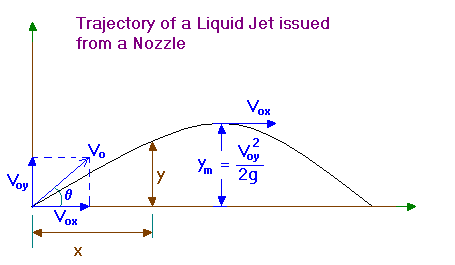### Trajectory of a free jet

Home -> Lecture Notes -> Fluid Mechanics -> Unit-II

In a free jet the pressure is atmospheric throughout the trajectory.Vox = Vo cosq = constant = Vx

Voy = Vo sinq

x = Vox t

y = Voy t – gt2/2

eliminating t gives,

y = x Voy/Vox – gx2/(2Vox2)

i.e,

y = x tanq – gx2/(2Vo2 cos2q)

This is the equation of the trajectory.

---------------

At the point of maximum elevation, Vy = 0 and application of Bernoulli’s law between the issue point of jet and the maximum elevation level,

Vo2/(2g) = Vox2/(2g) + ym

Since, Vo2/(2g) = Vox2/(2g) + Voy2/(2g)

we get,

ym = Voy2/(2g)